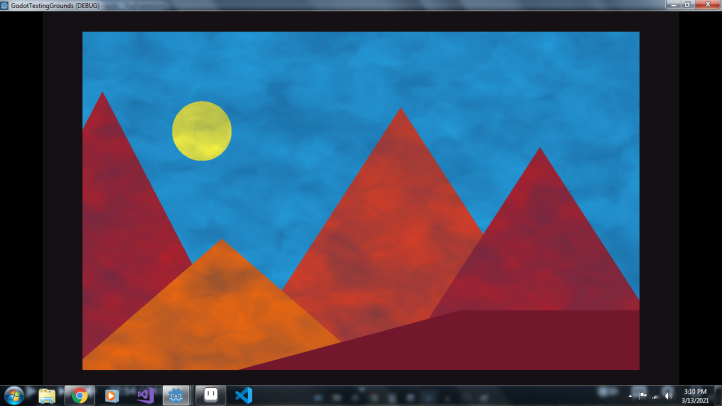A simple shader that outputs 3 shapes : A quadrilateral( square/rect ), A Circle, A Polygon (Triangle to Infinitygon).

This shader can be used in fast level designing such as the shapes available on unity. The cover image is an example of a scene i designed with this shader, it took 5 minutes.

The parameters are :

Shape – (0, 1, 2) – 0 is Circle, 1 is Rect, 2 is Polygon

Poly Count – (3 – inf) – 3 is Triangle, 4 is Square, 5 is Pentagon and so on. (Only works if shape is a Polygon)

(Ignore the fog in the cover photo :/)

``````shader_type canvas_item;

uniform int shape = 0;
uniform float poly_count = 3.0;

const float PI =  3.14159265359;
const float TWO_PI =  6.28318530718;

float Circle(vec2 st)
{
vec2 center = vec2(0.5) - st;
return step(length(center), 0.5);
}

float Square(vec2 st)
{
return 1.0;
}

float Triangle(vec2 st, float width)
{
st = st * 2.0 - 1.0;

if (poly_count > 2.0)
{
if (poly_count < 4.0)
{
st.y += 0.25;
}
}

float angle = atan(st.x, st.y) + radians(180.0);
float radius = TWO_PI / float(poly_count);

float dist = cos(floor(0.5 + angle / radius) * radius - angle) * length(st);
float poly = step(width, dist);
return 1.0 - poly;
}

void fragment()
{
vec2 st = UV;
st.y = 1.0 - st.y;

float sh;

if (shape == 0)
{
sh = Circle(st);
}
else if (shape == 1)
{
sh = Square(st);
}
else if (shape == 2)
{
sh = Triangle(st, 0.5);
}

vec3 color = vec3(sh);

color = 1.0 - color;
float alpha = 1.0 - color.r;
color = 1.0 - color;

COLOR = vec4(color, alpha);
}``````
###### Tags
2d, shapesThe shader code and all code snippets in this post are under CC0 license and can be used freely without the author's permission. Images and videos, and assets depicted in those, do not fall under this license. For more info, see our License terms.